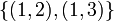# Generating sets for symmetric group:S3

## Contents

View generating sets for particular groups | View other specific information about symmetric group:S3

We take$S_3$ as the symmetric group acting on the set$\{ 1,2,3 \}$.

## List of generating sets

The minimum size of generating set is 2 and the maximum size of irredundant generating set is also 2. Some important generating sets are listed below.

Generating set Size of generating set Diameter Statistics for number of group elements for each minimum word length, starting from zero and going up to the diameter (must add up to 6) Other similar generating sets under conjugation More information$\{ (1,2), (2,3) \}$ 2 3 1,2,2,1$\{ (1,2), (1,3) \}$$\{ (1,3), (2,3) \}$
#Bruhat ordering$\{ (1,2), (1,3), (2,3) \}$ 3 2 1,3,2 none #Generating set of all transpositions$\{ (1,2), (1,2,3) \}$ 2 2 1,3,2$\{ (1,2), (1,3,2) \}$$\{ (1,3), (1,2,3) \}$$\{ (1,3), (1,2,3) \}$$\{ (1,3), (1,3,2) \}$$\{ (2,3), (1,2,3) \}$$\{ (2,3), (1,3,2) \}$
#Dihedral rotation and reflection generating set

## Generating set of all transpositions

Note that the left and right Cayley graphs are identical because the generating set is a conjugacy class of involutions. Also, we can unambiguously assign a direction (away from the identity) to each edge because there are no cycles of odd length, which in turn follows from the fact that all the generators are odd permutations.

## Bruhat ordering

The symmetric group of degree three can be viewed as a Coxeter group, with generators$s_1 = (1,2)$ and$s_2 = (2,3)$. The presentation is:$\langle s_1, s_2 \mid s_1^2 = e, s_2^2 = e, (s_1s_2)^3 = e \rangle$.

We can thus consider a Bruhat ordering on the elements of the symmetric group of degree three. Note that the Bruhat ordering depends on the specific choice of transpositions we use to generate the group, which in turn depends on an implicit order of the elements$\{ 1,2,3 \}$ that the group acts on (up to reversal). Thus, the Bruhat ordering is not invariant under conjugation.

The Bruhat ordering on the symmetric group of degree three has the special feature (no longer true for higher degree) that any two elements with distinct Bruhat lengths are comparable in the order. In the Bruhat ordering, there are four levels based on Bruhat length:

Length Number of elements of that length Elements of that length Conjugacy class information for these elements
0 1$()$ -- the identity element a single conjugacy class
1 2$\! s_1 = (1,2)$ and$\! s_2 = (2,3)$ all the elements are conjugate but do not form a complete conjugacy class
2 2$\! s_1s_2 = (1,2,3)$ and$\! s_2s_1 = (1,3,2)$ the elements form a single conjugacy class
3 1$\! s_1s_2s_1 = (1,3)$ a single element, part of a conjugacy class whose other elements have length 1

The element of length$3$, is, in matrix terms, the antidiagonal matrix:$\begin{pmatrix} 0 & 0 & 1 \\ 0 & 1 & 0 \\ 1 & 0 & 0 \\\end{pmatrix}$